## 9. Particle in a one-dimensional finite depth square well.

Now we will consider a one-dimensional field in which the potential V is everywhere 0 except on the interval [0;L] where the potential energy of a particle is negative with constant value U0 < 0. Intervals like this are said one-dimensional potential wells with depth U0.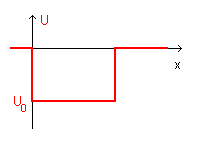In Classical Mechanics a particle with positions 0<x<L and total energy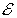lesser than 0 but greater than U0 can not escape from the well because outside it its kinetic energy K =-0 would be negative.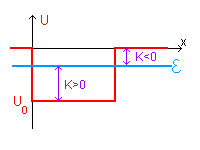The Quantum Mechanics approach is the following:

• if 0<x<L the wave function must be a solution of the equation (the subscript I stands for 'Internal')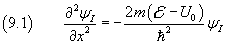• if x<0 or x>L the wave function must be a solution of the equation (the subscript E stands for 'External')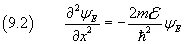Since the wave function must be continuous and differentiable in the entire field, for x=0 or x= L the two solutions and their derivatives must have the same values.

Moreover, since the Quantum Mechanics approach must asymptotically converge to Classical Mechanics, x→±∞ ⇒ ψ → 0.

• With a method similar to that taken in the previous section 8, we assume for the equation (9.1) a solution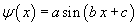and, in the same way we obtain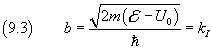But in this case, we cannot assume the boundary conditions ψ(0)=0 e ψ(L)=0, because the walls of the pit have finite altitude. So, for now, we have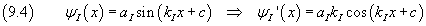• Solutions of (9.2) can not be something like, because the second derivative implies a negative value to the the square of b. If we otherwise assume solutions like ψ(x) = a eb x, differentiating twice with respect to x we have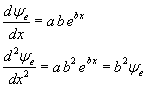and the comparison with (9.2) givesThis equality is acceptable because, by hypothesis,is negative. Therefore outside the well the function may have the form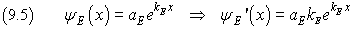• Since the wave function must be zero for x→±∞, we assume (the subscript EL stands for 'External Left' and the subscript ER stands for 'External Right' )

• for x<0: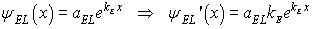• for x>L: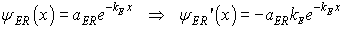For x=0 the two solutions and their derivatives must be equal. So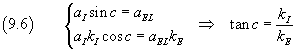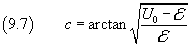The same happens for x=L. So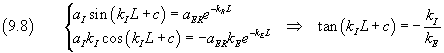From (9.6) and (9.8) we have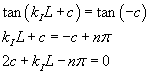Finally, using the values of c and kI from (9.3) and (9.7), we have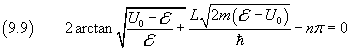By solving this equation with respect tofor each value of n we can obtain the energy levels inside the well. There is no analytical way to calculate thise solutions, but they can be approximated at will with a numerical method beginning from n=1 and raising it while negative solutions are found.

Here there is a Javascript function that calculates energy levels of an electron in a one-dimensional well, given its width, measured in nanometers, and its depth measured in volts.

JS uses the simple bisection algorithm. The code is in the HTML source of this page.